# DAV Class 7 Maths Chapter 11 Brain Teasers Solutions

The DAV Maths Class 7 Solutions and DAV Class 7 Maths Chapter 11 Brain Teasers Solutions of Perimeter and Area offer comprehensive answers to textbook questions.

## DAV Class 7 Maths Ch 11 Brain Teasers Solutions

Question 1.
A. Tick (✓) the correct option:
(i) The diameter of a circle whose circumference is 22 cm is —
(a) 3.5 cm
(b) 7 cm
(c) 14 cm
(d) 12 cm
(b) 7 cm

Circumference of circle = 22 cm
∴ 2πr = 22
⇒ 2 × $$\frac{22}{7}$$ × r = 22
⇒ r = $$\frac{7 \times 22}{2 \times 22}$$
⇒ r = $$\frac{7}{2}$$ = 3.5 cm
∴ Diameter of the circle = 2r
Hence, (b) is the correct option.

(ii) Area of a triangle whose base is 3 cm and height is 7 cm is —
(a) 10.5 cm2
(b) 21 cm2
(c) 10.5 cm2
(d) 105 cm2
(a) 10.5 cm2

Here, base of traingle = 3 cm
Height of triangle = 7 cm
∴ Area of traingle = $$\frac{1}{2}$$ × Base × Height
= $$\frac{1}{2}$$ × 3cm × 7cm
= $$\frac{21}{2}$$ cm2
= 10.5 cm2
Hence, (a) is the correct option.

(iii) Area of afield is 500000 m2. Its area in hectare is
(a) 500 hectare
(b) 50 hectare
(c) 5 hectare
(d) 5000 hectare
(b) 50 hectare

Area of field = 500000 m
and 1 hectare = 10000 m
∴ Area of field in hectage = $$\frac{500000}{10000}$$ hectare
= 50 hectare
Hence, (b) is the correct option

(iv) Which of the following is the circumference of a circle?
(a) 2πr2
(b) πd
(c) πr2
(d) 2πd
(b) πd

Circumference of a circle = 2πr
⇒ Circumference of a circle = π(2r)
Circumference of a circle = πd [∵ Diameter = 2 radius]
Hence, (b) is the correct option.

(v) Area of a right angled triangle whose sides containing the right angle are 12 dm and 5 dm is
(a) 60 dm2
(b) 30 dm
(c) 60 dm
(d) 30 dm2
(d) 30 dm2

Let the base of right triangle = 12 dm
and height of right triangle = 5 dm
∴ Area of right angled triangle = $$\frac{1}{2}$$ × Base × Height
= $$\frac{1}{2}$$ × 12 dm × 5 dm
= 30 dm2
Hence, (d) is the correct answer.(i) Find the area of a parallelogram whose base is 14 cm and height is 5 cm.
Base of parallelogram = 14 cm
Height of parallelogram = 5 cm
Area of parallelogram = Base × Height
= 14 cm × 5 cm
= 70 cm2
∴ Area of parallelogram is 70 cm2.

(ii) Find the height of a rhombus whose area is 168 dm2 and the corresponding base is 21 dm.
Here, base of rhombus = 21 dm
Area of rhombus = 168 dm2
Height of rhombus = ?
Area of rhombus = Base × Height
⇒ 168 dm2 = 21 dm × Height
⇒ Height = $$\frac{168 \mathrm{dm}^2}{21 \mathrm{dm}}$$ = 8 dm
Hence, the height of rhombus is 8 dm

(iii) A 44 cm long wire is bent to form a circle. Find the diameter of the circle.
Let the radius of the circle be r cm.
According to the question, we have
Circumference of the circle = Length of the wire
∴ 44 cm = 2πr
= 44 = 2 × $$\frac{22}{7}$$ × r
⇒ r = $$\frac{44 \times 7}{2 \times 22}$$
⇒ r = 7
But, diameter of the circle = 2r = 2(7)
= 14 cm
Hence, the diameter of the circle is 14 cm.

(iv) Find the area of a circle whose circumference is 132 cm.
Let the radius of the circle be r cm
Circumference of the circle = 132 cm
⇒ r = $$\frac{7 \times 132}{2 \times 22}$$
⇒ r = $$\frac{924}{44}$$
⇒ r = 21 cm
Now, area of circle = πr2
= $$\frac{22}{7}$$ × (21)2 cm2
= $$\frac{22}{7}$$ × 21 × 21 cm2
= 22 × 3 × 21cm2
= 1386 cm2
Hence, the area of the circle is 1386 cm2.

(v) Find area of a rhombus whose one side is 8 cm and height is 0.8 dm.
Here, base of rhombus = 8 cm
Height of rhombus = 0.8 dm = 0.8 × 10 cm
= 8 cm
∴ Area of rhombus = Base × Height
= 8cm × 8cm = 64 cm2
Hence, the area of rhombus is 64 cm2.

Question 2.
A path 3.5 m wide runs inside along the boundary of a square field whose side is 65 m. Find the area of the path. Also find the cost of manuring the rest of the field at the rate of ₹ 25 per square meter.Area of the square = (side)2
= (65)2 = 4225 m2

Side of the inner square field
= 65 – 2 × 3.5
= 65 – 7 = 58 m
Area of the inner square field = (58)2 = 3364 m2
Area of the path = 4225 – 3364 = 861 m2
Cost of manuring the inner field = 3364 × 25 = ₹ 84,100
Hence the required area = 861 m2 and the required cost;
= ₹ 84,100.Question 3.
A rectangular field has 80 m length and 48 m breadth. Three roads, each of width 2 m pass through the field such that two roads are parallel to the breadth and the third road parallel to the length. Find the area covered by the roads.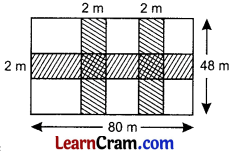= 2 × (48 × 2)
= 192 m2
Area of the road parallel to the length
= 80 × 2
= 160 m2
Area of the common portion = 2 × 2 + 2 × 2
= 8m2
Total area of the three roads = 192 m2 + 160 m2 – 8 m2
= 344 m2
Hence the required area = 344 m2

Question 4.
A design on the wall of a room consists of 1000 tiles of the shape of parallelogram. If altitude and base of tiles are 10 cm and 4 cm respectively, find the cost of polishing the design at the rate of ₹ 9.50 per dm2.
Area of one tile = base × altitude
= 10 × 4 = 40 cm2

Area of 1000 tiles = 40 × 1000 = 40000 cm2 = ₹ 3800
= $$\frac{40000}{100}$$dm2
= 400 dm2
∴ Cost of polishing the tiles = 400 × 9.50
Hence the required cost = ₹ 3800.

Question 5.
In the given figure, PQ ∥ SR, SP ⊥ PQ, PQ = 60 cm, SR = 36 cm and QR = 25 cm. Find the area of the quadrilateral PQRS.Draw RT ⊥ PQ
∴ TQ = PQ – PT
= PQ – SR
= 60 – 36 = 24 cm

In ΔRTQ, ∠RTQ = 90°
RT2 + TQ2 = QR2 (By Pythagoras Theorem)
RT2 + (24)2 = (25)2
RT2 + 576 = 625
RT2 = 625 – 576
RT2 = 49
RT = 7 cm
Now area of quadrilateral PQ RS = $$\frac{1}{2}$$ (sum of parallel sides) × altitude
= $$\frac{1}{2}$$ (60 + 36) × 7
= $$\frac{1}{2}$$ × 96 × 7
= 48 × 7
= 336 cm2
Hence the required area = 336 cm2.

Question 6.
There is a circular pond and footpath runs along its boundary. A man walks around it, exactly once, keeping close to the edge. If his step is 44 cm long and he takes exactly 600 steps to go around the pond, what is the radius of the pond?
Distance travelled by man around the edge of the pond is 600 steps
= 600 × 44 = 26400 cm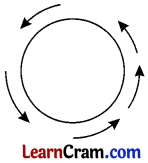Circumference of the pond = 26400 cm
2πr = 26400
2 × $$\frac{22}{7}$$ × r = 26400
r = $$\frac{26400 \times 7}{2 \times 22}$$
r = 4200 cm = 42 m
Hence the required radius = 42 m.

Question 7.
From a square metal sheet of side 28 cm, a circular sheet is cut off. Find the radius of the largest possible circular sheet that can be cut. Also find the area of the remaining sheet.
Side of the square sheet = 28 cm
∴ Its area = (28)2 = 784 cm2
Radius of the largest circular sheet = $$\frac{28}{2}$$ = 14 cm
∴ Area of the circular sheet = πr2 = $$\frac{22}{7}$$ × 14 × 14 = 616 cm
Area of the remaining sheet = 784 – 616 = 168 cm2
Hence the required radius is 14 cm and area = 168 cm2.Question 8.
Triangle ABC is right angled at A. AD is drawn perpendicular to BC. If AB = 5 cm and AC = 12 cm, find the area of ΔABC. Also find the length of AD.
Here, base AB = 5 cm and altitude AC = 12 cm
Hence, the area of ΔABC is 30 cm2 and the length of AD is 4.615 cm.
∴ Area of ΔABC = $$\frac{1}{2}$$ × AB × AC
= $$\frac{1}{2}$$ × 5 × 12
= 30 cm2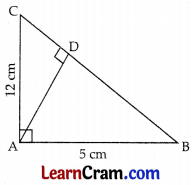Now, in ΔABC, we have
(BC)2 = (AB)2 + (AC)2 [BY Pythagoras theorem]
⇒ (BC)2 = (5)2 + (12)2
⇒ (BC)2 = 25 + 144
⇒ (BC)2 = 169
⇒ BC = 13 cm
Also, Area of ΔABC = $$\frac{1}{2}$$ × base BC × Altitude AD
⇒ 30 = $$\frac{1}{2}$$ × 13 × AD
⇒ AD = $$\frac{30 \times 2}{13}$$
⇒ AD = $$\frac{60}{13}$$
= 4.615 cm

## DAV Class 7 Maths Chapter 11 Value Based Questions

Question 1.
Find the area of a triangle whose sides are in the ratio 5 :12 :13 and its perimeter is 60 cm.
Let the sides of the triangle be 5x, 12x and 13x.
Perimeter of triangle = 60 cm
∴ 5x + 12x + 13x = 60 cm
⇒ 30x = 60
⇒ x = $$\frac{60}{30}$$
⇒ x = 2
So the sides of the triangle are 5(2), 12(2), 13(2), i.e.; 10 cm, 24 cm and 26 cm.
Now, (10)2 + (24)2
= 100 + 576 = 676
= (26)2
So, the triangle is a right angled triangle as the square of one side of a triangle is equal to the sum of the squares of the other two sides. And, hypotenuse of the triangle is of length 26 cm.
∴ Area of triangle = $$\frac{1}{2}$$ × Base × Height
= $$\frac{1}{2}$$ × 10 × 24 = 5 × 24
= 120 cm2
Hence, the area of the triangle is 120 cm2.

Question 2.
AD is the diameter of a circle of radius 6 cm and AB = BC = CD. Semi-circles are drawn with AB and BD as diameters as shown in the figure.
Find the perimeter and area of the shaded region.Here, AD = 2 × 6 cm = 12 cm
AB = BC = CD = $$\frac{1}{3}$$AD
⇒ AB = BC = CD = $$\frac{1}{3}$$(12) cm
⇒ AB = BC = CD = 4 cm
BD = BC + CD = 4 cm + 4 cm = 8 cm
Perimeter of shade region = (circumference of semi-circle with diameter AD)+(circumference of semi-circle with diameter AB) + (circumference of semi-circle with diameter BD)
Area of the shaded region = (Area of semi-circle with diameter AB) + (Area of semi-circle with diameter AD) – (Area of semi-circle with diameter BD)
= π(2)2 + π(6)2 – π(4)2
= π(4) + π(36) – π(16)
= π(4 + 36 – 16)
= π(4 + 20)
= 24π
= 24 × $$\frac{22}{7}=\frac{528}{7}$$
= 75.428 cm2
Hence, the perimeter and area of the shaded region are 37.714 cm and 75.428 cm2 respectively.

Question 3.
Four equal circles are described about four corners of a square so that each touches the two of the others. Find the area of the part of the square enclosed by the circles if the side of the square is 14 cm.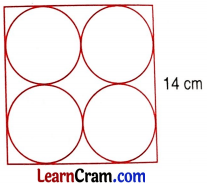From the figure, radius of each circle = 3.5 cmArea of the part of the square enclosed by the circles = Area of the shaded region
∴ Area of the shaded region = Area of square – Area of four circles
= (Side)2 – 4(πr2)
= (14)2 – 4[π(3.5)2]
= 196 – 4($$\frac{22}{7}$$ × 3.5 × 3.5)
= 196 – 4($$\left(\frac{269.5}{7}\right)$$)
= 196 – 4(38.5)
= 196 – 154
= 42 cm2
Hence, the area of the part of the square enclosed by the circles is 42 cm2.

## DAV Class 7 Maths Chapter 11 Enrichment Questions

Question 1.
A regular hexagonal picture has 4 cm long side. Find the cost of lace needed to make the border for this picture if the lace costs ₹ 2.50 per cm.
Perimeter of the regular hexagonal picture = 4 × 6 = 24 cm
∴ Cost of lace = 24 × 2.50 = ₹ 60
Hence the required cost = ₹ 60.

Question 2.
Find the area of unshaded portion of given figure, shaded portions being semi-circular regions.Area of the rectangle = 14 × 10 = 140 m2
Diameter of one semi-circle = $$\frac{14}{2}$$
∴ its radius = $$\frac{7}{2}$$
Area of one semi-circular region = $$\frac{1}{2}$$r2
Area of the two semi-circular region = 2 × $$\frac{1}{2}$$πr2
= πr2
= $$\frac{22}{7} \times \frac{7}{2} \times \frac{7}{2}=\frac{77}{2}$$m
= 38.5 m2
Area of the unshaded region = 140 – 38.5 = 101.5 m2
Hence the required area = 101.5 m2.

Question 3.
Four cows are tethered at four corners of a square field of 70 m side as shown in given figure so that they can reach each other. What area of the field will remain ungrazed?Radius of each quarter = $$\frac{70}{2}$$ = 35 m
Area of one quarter = $$\frac{1}{4}$$πr2
Area of the four quarter = 4 × $$\frac{1}{4}$$πr2
= πr2
= $$\frac{22}{7}$$ × 35 × 35
= 3850 m2
Area of the square field = 70 × 70
= 4900 m2
Area of the ungrazed portion = 4900 – 3850
= 1050 m2
Hence the required area = 1050 m2.Question 1.
The area of a circle is 616 cm2, find its radius.
Area of circle = 616 cm2
πr2 = 616
$$\frac{22}{7}$$ × r2 = 616
r2 = 616 × $$\frac{7}{22}$$
r2 = 28 × 7
r2 = 4 × 7 × 7
r = 2 × 7 = 14 cm
Hence the required radius = 14 cm.

Question 2.
A circular park of diameter 70 m is surrounded by a walking track of width 1.4 m. Find the area of the track. Also find the cost of constructing the track at ₹ 15 per m2.
The diameter of the park = 70 m
∴ Its radius = $$\frac{70}{2}$$ = 35 m
Area of the park = πr2
= $$\frac{22}{7}$$ × 35 × 35
= 3850 m2
Width of the track = 1.4 m
∴ Radius of the park including track = 35 + 1.4 = 36.4 m
∴ Its area = πr2
= $$\frac{22}{7}$$ × 36.4 × 36.4
= 4164.16 m2
Area of the track = 4164.16 – 3850 = 314.16 m2
Cost of constructing the track = 314.16 × 15 = ₹ 4712.40
Hence the required cost = ₹ 4712.40.

Question 3.
Semi-circular parks are made with all sides of a square field with side 14 m. Find the area of the 4 semi-circular parks.Diameter of each semi-circle = 14 m
∴ Radius of each semi-circular park = $$\frac{14}{2}$$ = 7 m
Area of one semi-circle = $$\frac{1}{2}$$πr2
∴ Area of the four semi-circles = 4 × $$\frac{1}{2}$$πr2
= 2πr2 = 2 × $$\frac{22}{7}$$ × 7 × 7 = 308 m2
Hence the required area = 308 m2.

Question 4.
In the given figure the measurement are g< area of the shaded region.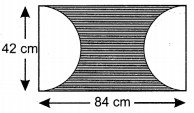Area of the rectangle
= 84 × 42
= 3528 cm2
Radius of one semi-circle = $$\frac{42}{2}$$ = 21 cm
Area of the two semi-circle = 2 × $$\frac{1}{2}$$πr2
= πr2
= $$\frac{22}{7}$$ × 21 × 21
= 1386 cm2
∴ Area of the shaded portion = 3528 – 1386
= 2142 cm2
Hence the required area = 2142 cm2.Question 5.
A wire of length 22 m is to be bent in the form of a circle and then in the form of a square. Find the difference between the areas of the two figures.Circumference of the circle = 2πr
2πr = 22
2 × $$\frac{22}{7}$$ × r = 22
Area of the circle = πr2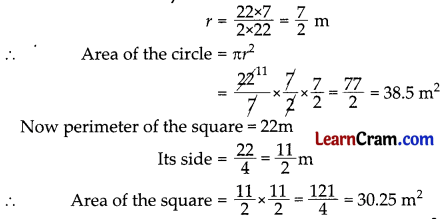Difference between the two areas = 38.5 – 30.25 = 8.25 m2
Hence the required area = 8.25 m2.

Question 6.
A wire is in the form of a circle of radius 21 m. It is bent into an equilateral triangle. Find the area of the triangle.
Circumference of the circle = 2πr = 2 × $$\frac{22}{7}$$ × 21 = 132 m
Perimeter of equilateral triangle = 132 m
Its side = $$\frac{132}{3}$$ = 44m
Area of the equilateral triangle = $$\frac{\sqrt{3}}{4}$$ × 44 × 44 = 484√3 m2
Hence the required area = 484√3 m2.

Question 7.
Measurements are given in the figure. Find the area of the quadrilateral ABCD.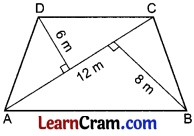Area of ΔABC = $$\frac{1}{2}$$ × 12 × 8 = 48 m2
Area of ΔADC = $$\frac{1}{2}$$ × 12 × 6 = 36 m2
Total area of □ ABCD = 48 + 36 = 84 m2
Hence the required area = 84 m2.

Question 8.
The perimeter of a rhombus is 28 m. If the perpendicular distance between the opposite parallel sides is 5 m, find the area of the rhombus.
Perimeter of the rhombus = 28 m
Its side = $$\frac{28}{4}$$ = 7 m
Area of rhombus = base × altitude
= 7 × 5 = 35 m2
Hence the required area = 35 m2.

Question 9.
The length of the two diagonals of a rhombus are 8 cm and 6 cm respectively. Find the perimeter and area of the rhombus.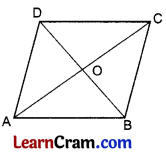Here AC = 8 cm ∴ OA = 4 cm
BD = 6 cm OB = 3 cm
∵ In right ΔAOB,
OA2 + OB2 = AB2 (By Pythagoras Theorem)
(4)2 + (3)2 = AB2
16 + 9 = AB2
25 = AB2
∴ AB = 5 cm
Perimeter of the rhombus = 5 × 4 = 20 cm
Area of the rhombus = $$\frac{1}{2}$$ × d1 × d2
= $$\frac{1}{2}$$ × 8 × 6 =24 cm2
Hence the required perimeter is 20 cm and the area is 24 cm2.Question 10.
The area of a rhombus is 105 cm2. If its altitude is 7 cm, find its perimeter.
Area of the rhombus = base x altitude
105 = base × 7
base = $$\frac{105}{7}$$ = 15 cm
The perimeter of the rhombus = 15 × 4 = 60 cm
Hence the required perimeter = 60 cm.

Question 11.
The diagonals of a rhombus are 24 cm and 10 cm respectively. Find its area and the perimeter.Area of the rhombus = $$\frac{1}{2}$$ × d1 × d2
= $$\frac{1}{2}$$ × 24 × 10 = 120 cm2
AB2 = OA2 + OB2
AB2 = (12)2 + (5)2
= 144 + 25
= 169
∴ AB = 13 cm
Perimeter of the rhombus = 13 × 4 = 52 cm
Hence the required area = 120 cm2 and perimeter = 52 cm.

Question 12.
The base of the triangular field is twice its altitude. If its area 256 m2, find its base.
Let the altitude of the field be x m
its base = 2x m
∴ Area of the triangular field = $$\frac{1}{2}$$ × base × attitude
256 = $$\frac{1}{2}$$ × x × 2x
256 = x2
∴ x = $$\sqrt{256}$$ = 16 m
∴ Base of the triangular field = 16 × 2 = 32 m
Hence the required base = 32 m.

Question 13.
A square is drawn on the hypotenuse of an isosceles right triangle of side 6 cm. Find the area and perimeter of the square.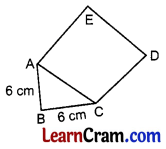In right ΔABC,
AB2 + BC2 = AC2
(6 )2 + (6)2 = AC2
36 + 36 = AC2
72 = AC2
AC = $$\sqrt{72}$$ = 6√2 cm
∴ Perimeter of the square ACDE = 4 × 6√2 = 24√2 cm
Area of the square ACDE = (6√2)2 = 72 cm2
Hence the required perimeter is 24√2 cm and the area is 72 cm2.Question 14.
Two roads of width 2.5 m are constructed in a rectangular park, one is parallel to length and the other parallel to its breadth. Find the cost of constructing the roads at ₹ 5 per m2 if the length and breadth of the park are 36 m and 24 m respectively.
Area of the road parallel to the length = 36 × 2.5 = 90 m2
Area of the road parallel to the breadth = 24 x× 2.5 = 60 m2
Area of the common region = 2.5 × 2.5 = 6.25 m2
∴ Total area of the roads = 90 + 60 – 6.25
= 150 – 6.25 = 143.75 m2 and cost of construction = 5 × 143.75 = ₹ 718.75
Hence the required area = 143.75 m2 and the cost of construction = ₹ 718.75.

Question 15.
12000 tiles of measuring 10 cm by 8 cm are used to cover the surface of a hall. Find the area of the surface of the hall. If 8 m is the length of the hall, find its breadth.
Area of 1 tile = 10 × 8 = 80 cm2
Area of 12000 tiles = 80 × 12000
= 960000 cm2
= 96 m2
Breadth of the hall = 96 ÷ 8 = 12 m
Hence the area of the surface is 96 m2 and the breadth is 12 m.

Question 16.
Find the area of the shaded region in given figure.Area of the rectangle = 28 × 18 = 504 m2
EF = 28 – 2 × 3 = 22 m
FG = 18 – 2 × 3 = 12 m
∴ Area of the rectangle EFGH = 22 × 12 = 264 m2
Area of the shaded region = 504 – 264 = 240 m2
Hence the required area = 240 m2.

Question 17.
Swati draws the figure of an aeroplane as shown in the given figure. Here, the wings ABCD and GHIF form two parallelograms respectively. The tail DEF is an equilateral triangle, the cockpit CKI is a semi-circle and middle part DCIF is a square. Calculate the area of the plane figure if BP ⊥ CD and HQ ⊥ FI. The measurements in the figure are in cm.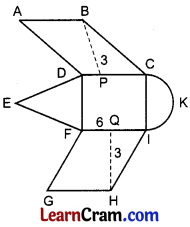Area of equilateral triangle = $$\frac{1}{2}$$πr2
= $$\frac{\sqrt{3}}{4}$$ × 6 × 6 = 9√3 cm2